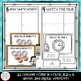# Second Grade Math Centers BundleSubject
Grade Levels
Resource Type
Common Core State Standards
Product Rating
File Type

Compressed Zip File

Be sure that you have an application to open this file type before downloading and/or purchasing.

373 MB|400 pages
Share
9 Products in this Bundle
9 products
Bonus
1 file
Math Center Teacher Tips and Tricks
Bundle Description

This 2nd grade bundle includes hands-on and engaging math centers for the entire year!!! You will be getting a total of 90 math centers!

Each set of centers has the same format, so students will learn the expectations and procedures and then be able to complete centers for the entire year without many new directions.

The centers are engaging and include sorts, task cards, math writing, color coding, matching, etc. Each set of centers has a recording book that students use for all 10 centers in that unit.

This resource was created in collaboration by Third Grade Pad and Not So Wimpy Teacher. Creating this resource as a team helped us to increase the quality of the math centers!

**Would you like to learn how I use these math centers? Click HERE to view a free video on how to implement math centers.**

Centers are included in color and black line for your convenience.

JUST ADDED! This bundle now includes a 40 page document with math center tips, schedules, posters and labels!

******************************************

Centers Included:

Back to School

1. Math journaling (Subtraction)

2. Word Problems (Addition & Subtraction)

3. Roll a Problem (Place Value & Making Numbers)

4. Making Numbers (10 more and 10 less)

5. 2- Digit Addition

6. Making 10

7. Measuring (with non-standard units)

8. Missing Numbers (additions and subtraction)

9. Telling Time

10. Comparing Numbers (2-Digit)

Place Value

1. Math journaling (counting 10s)

2. Word Problems (Addition & Subtraction)

3. Roll a Problem (3-Digit Place Value & Making Numbers)

4. Odds & Evens

5. Adding to Subtract

6. Making 10

7. Counting On (counting by 1s, 2s and 3s)

8. Number Patterns

9. Place Value Match (Expanded form and Base 10 Models)

10. Comparing Numbers (3 digit)

2 Digit Addition

1. Math journaling (missing addend)

2. Word Problems (with and without regrouping, two and three addends)

3. Roll a Problem

4. Three & Four Addends

5. Using Expanded Form

6. Using Break Apart Strategy

7. Spin to Add (with and without regrouping)

8. Missing Numbers (using a hundreds chart to add)

9.Addition and Sum Matching (with and without regrouping)

10. Using a Number Line to Add

2 Digit Subtraction

1. Math journaling

2. Word Problems (with and without regrouping)

3. Roll a Problem

4. Choose a Strategy

5. Using Friendly Numbers

6. Using Break Apart Strategy

7. Spin to Subtract (with and without regrouping)

8. Missing Numbers (using a hundreds chart to subtract)

9. Subtraction Fact and Difference Matching

10. Using a Number Line to Subtract

Time and Money

1. Math journaling (adding coins)

2. Word Problems (money and change)

3. Roll a Problem (coin value)

4. Drawing clocks

5. Determining the minutes past the hour or until the next hour

6. Identifying coin value

7. Spin to Make Change

8. True of False (Does the digital time match the analog clock?)

9. Reading Analog Clocks

10. Reading clocks and AM or PM

3-Digit Addition

1. Math journaling (with regrouping)

2. Word Problems (with and without regrouping)

3. Roll a Problem

4. True or False Sort (checking answers)

5. Expanded Form to Add

6. Break Apart Strategy to Add

7. Spin to Add

8. Missing Numbers in Addition Equations

9. Equation and Sum Match

10. Number Lines to Add

3-Digit Subtraction

1. Math journaling (with regrouping)

2. Word Problems (with and without regrouping)

3. Roll a Problem

4. True or False Sort (checking answers)

5. Add to Subtract

6. Break Apart Strategy to Subtract

7. Spin to Subtract

8. Missing Numbers in Subtraction Equations

9. Equation and Difference Match

10. Number Lines to Subtract

Measurement & Graphs

1. Math journaling

2. Word Problems (addition & subtraction with measurement)

3. Building a Picture Graph

4. True or False Sort (data from a bar graph)

5. Line Plot Graph

6. Picture Graph Data

7. Measuring (inches and centimeters)

8. Build a Bar Graph

9. Estimate Measurement (with different units)

10. How much longer? (measuring two objects and comparing)

Geometry and Fractions

1. Math journaling

2. Drawing 2-D Figures

3. Making Shapes with Pattern Blocks

4. Naming the Fraction of a Whole

5. Making Rows and Columns

6. Attributes of Figures True/False Sort (2d and 3D)

7. Spin to Divide Shapes into Equal Shares

8. Missing Numbers (attributes of shapes)

9. Matching 2D and 3D Shapes with their Name

10. Adding Rows and Columns

Looking for these centers for another grade level?

Third Grade

Fourth Grade

Fifth Grade

Total Pages
400 pages
Answer Key
Included
Teaching Duration
1 Year
Report this Resource
Reported resources will be reviewed by our team. Report this resource to let us know if this resource violates TpT’s content guidelines.Sign Up